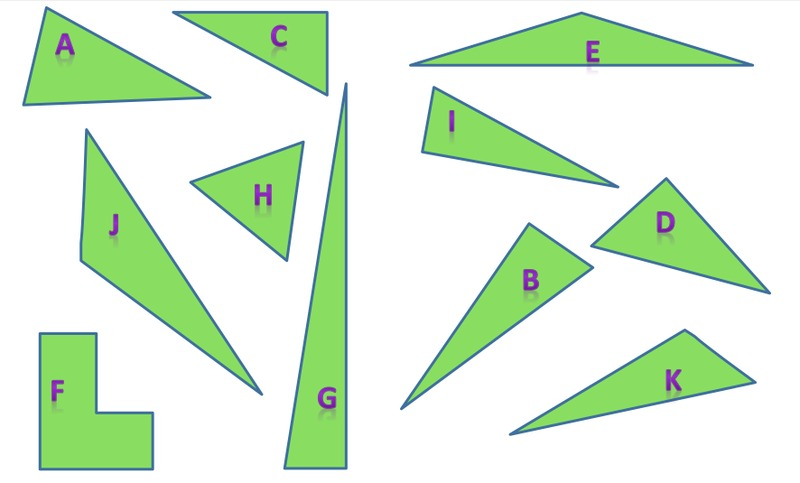# Are these right?

Alignments to Content Standards: 4.G.A.2

1. Which of the polygons are right triangles? Choose a measuring tool to help you determine this.These are right triangles These are not right triangles

2. For each right triangle, identify the right angle by marking it with a small square. Explain why the other polygons are not right triangles.

## IM Commentary

The purpose of this task is for students to measure angles and decide whether the triangles are right or not. Students should already understand concepts of angle measurement (4.MD.5) and know how to measure angles using a protractor (4.MD.6) before working on this task. Students should also understand that a defining attribute for a right triangle is that one of the three angles is 90 degrees. The task asks students to choose the appropriate tool which gives them a chance to decide for themselves what they will need to answer the question, thus allowing them to use appropriate tools strategically (MP5). The triangles in this task are smaller than most classroom protractors, so students have several choices. They might extend the sides of the triangle using a ruler. It might help them to cut out the shapes and place them on another piece of paper. A second and slightly more difficult method is to leave the polygons on the page and list the letters for the polygons in the appropriate entries of the table. For this students will need to manipulate the protractor or the orientation of the paper instead of rotating the shape itself, which can also serve a useful purpose later on. Alternatively, students might use the corner of a piece of paper that they have measured and know is 90 degrees, and then compare the angle formed by the edges of the paper with the angles in the triangles. The attached black-line master is for the version that encourages students to cut out and manipulate the polygons in ways that might make it easier to measure. An extension of this activity could be to have students classify the triangles in the right side of the chart based on other defining attributes (scalene, isosceles, equilateral, obtuse, acute).

The Standards for Mathematical Practice focus on the nature of the learning experiences by attending to the thinking processes and habits of mind that students need to develop in order to attain a deep and flexible understanding of mathematics. Certain tasks lend themselves to the demonstration of specific practices by students. The practices that are observable during exploration of a task depend on how instruction unfolds in the classroom. While it is possible that tasks may be connected to several practices, only one practice connection will be discussed in depth. Possible secondary practice connections may be discussed but not in the same degree of detail.

This particular task engages students in Mathematical Practice Standard 5, Use appropriate tools strategically. The task asks students to choose the appropriate tool which gives them a chance to decide for themselves what they will need to answer the question, thus allowing them to select appropriate tools. They may directly measure the angles of the triangles or may use a 90 degree template that they have constructed. As students become proficient in this practice, they will be able to consider a tool’s usefulness and consider its strengths and limitations, as well as know how to use it appropriately. The solution pathway that a student selects needs to make sense to him/her to be able to explain and justify (MP.3). Students will come to realize that certain methods/tools are more efficient and they will abandon less useful tools in favor of more appropriate strategies/tools.

This task was created as part of an Illustrative Mathematics Web Jam.

## Solution

1.2. Triangles B, C, D, G, and I each have one right angle.

Triangles A, K, J, H, and E do not have any right angles, but they are all triangles because they have three sides and three angles. F is not a triangle because it has 6 sides. It does have right angles.